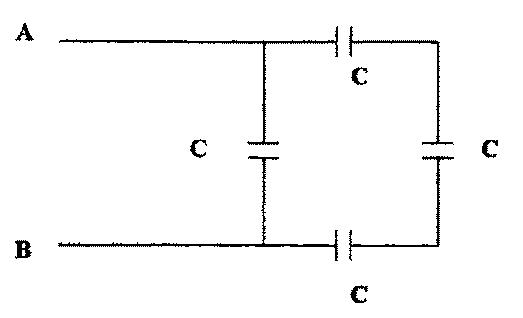## University of California, San Diego Physics 1b - Thermal Physics & Electromagnetism

 H. E. Smith Spring 2000Physics 1B - Tutorial #61. In the network above, each capacitor has a capacitance of 2µF.
• Calculate the equivalect capacitance of the network.

Ceq = C + C/3 = 2.67µF

• If 10V is applied across A and B, what is the charge on each capacitor?

Q(left) = CVAB = 2 x 10-5C
Q(top) = Q(right) = Q(bottom) CVAB/3 = 6.67 x 10-6C2. A capacitor made of a close pair of conducting plates, as shown above, is charged then discharged to produce a spark. The plates are charged a second time, and this time the plates are pulled further apart before being discharged. Will the second charge be (a) bigger than, (b) the same as, or (c) smaller than the first spark. Explain your reasoning.

The charge remains the same, but you are doing work pulling the plates apart, increasing the potential energy stored in the capacitor, PE = Q2/2C, where C =A/l has decreased becaused "l" has increased. Same charge, greater energy --> "bigger" spark.

3. A mountain lake is to be used as the water supply for an alpine village which lies a vertical distance h below the lake. The lake and village are to be connected by a system of pipes. How will the flow of water depend upon:
• the height, "h" of the lake above the village.
If "h" is greater the flow of water will be greater; The change in PE is greater so the velocity will be greater. Remember Bernoulli?

• the diameter of the pipes.
The flow will go as the cross-sectional area of the pipes ~ d2

• the length of the pipes (assuming that there is some form of viscous drag - friction).
If the length of the pipes were increased, with the same vertical drop, then the flow of water would decrease because there would be greater drag.

What would happen if you put in two parallel pipes of the same length & diameter?
You would double the flow.

What would happen if you doubled the length of the pipe?
You would decrease the flow.

What does this have to do with electricity?

• Flow of water is analogous to electrical current.
• The height of the lake, "h" is analogous to the potential (energy) difference or voltage in a circuit.
• In a circuit resistance, R =l/A. "A" would be the cross-sectional area of the pipe; "l" the length.
• Two parallel pipes = two parallel wires or resistances.
• Doubling the length of the pipe is like doubling the length of wire or placing equal resistances in series.4. Above is a diagram of the electrical system of an automobile. Which swithces (A, B, C, D, E, F) must be closed in order to
• a. Blow the horn. - A & B.
• b. Turn on the headlights. - A & E.
• c. Turn on the taillights. - A, D & C.
• d. Turn on the parking lights. - A & D.
• e. Turn on the dome light. - A & F.
• f. When do the side marker lights go on? - when the headlights go on.
Note that the whole body of the car, including the engine is wired together as a common ground. (Hecht: Ch. 19 #23, p. 749)

Gene Smith